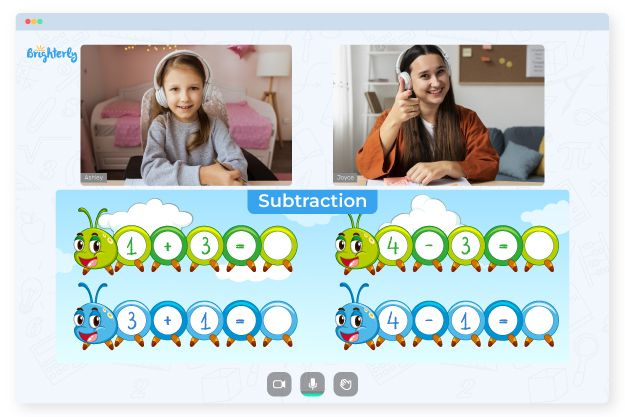# 3 Digit Subtraction With Regrouping Worksheets

Kids need to rearrange two or more numbers vertically to find the difference quickly. Taking a number from the next place value is known as regrouping or borrowing. Even in everyday life, subtracting and regrouping are critical concepts. So, kids need learning aids like 3-digit subtraction with regrouping worksheets to master math.

## Why to Use 3-Digit Subtraction with Regrouping Worksheets from Brighterly?

In mathematics, the term regrouping refers to transferring values from one place value to another. To regroup properly, students need to know place value. But what if they have a weak math foundation or have no prior knowledge of regrouping? A math tutor from Brighterly can help them understand the topic.

Frequently, kids get lost if tutors just give an overview of subtraction basics and jump into regrouping 3-digit numbers. Thus, the use of a subtract 3-digit numbers with regrouping worksheet may solidify students’ subtraction foundations. Tutors usually show pupils how to solve at least one of the worksheets’ problems before letting kids have a go.

Math for Kids

Is Your Child Struggling With Math?
1:1 Online Math Tutoring## How to Use Three-Digit Subtraction with Regrouping Worksheets in a Fun Way

Working with worksheets, kids start with a review of minuends, subtrahends, differences, and two-digit subtraction. You can use 3-digit subtraction with regrouping worksheets for 3rd grade students as a means of evaluation.### 3 Digits Subtraction Worksheets PDF

Three Digits Subtraction Worksheets### 3 Digits Subtraction Worksheets PDF

Subtraction 3 Digit Numbers Worksheet### 3 Digits Subtraction Worksheets PDF

Subtract 3 Digit Numbers With Regrouping Worksheet### 3 Digits Subtraction Worksheets PDF

3 Digit Subtraction Worksheets

Encourage kids to solve challenges independently and see how far they can go. If, in the end, students still experience difficulties, you can have them redo any or all of the preceding worksheets to refresh their memory on three-digit subtraction with regrouping.

### Subtraction Worksheets

Problems with Subtraction?• Is your child struggling with subtraction concepts?
• An online tutor could be the solution.

Does your child find the basics of subtraction to be challenging? Try learning with an online tutor.